#R语言字符串处理的一次经历

0
0
01. 云栖社区>
2. 博客>
3. 正文

# 1 数据描述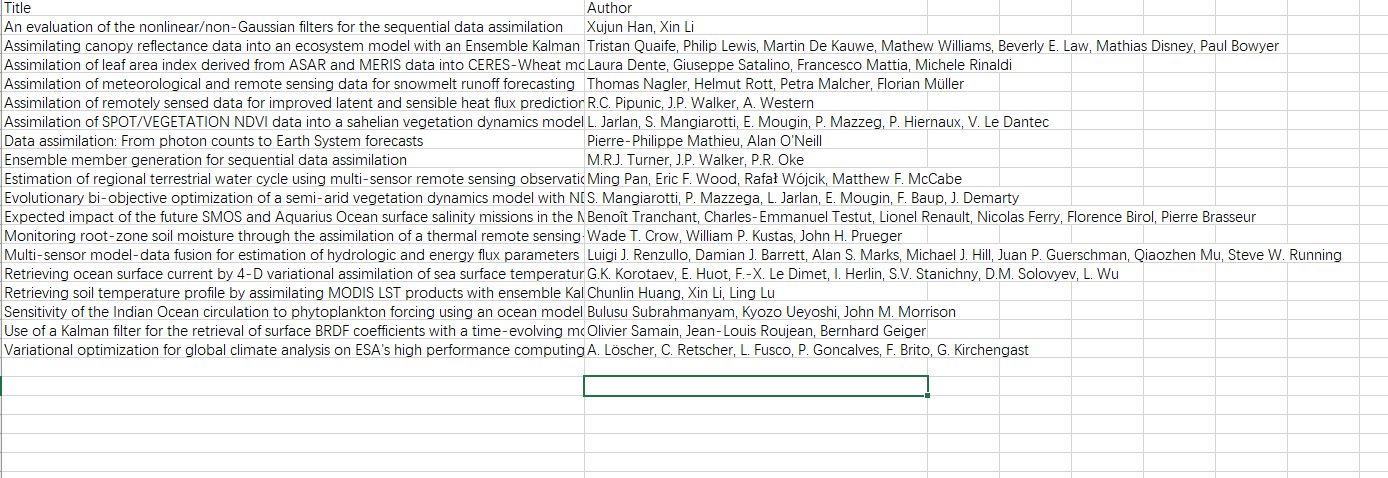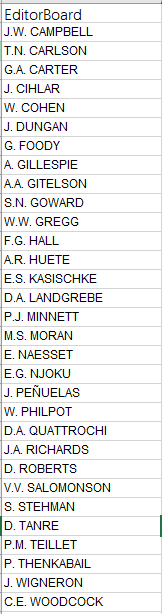# 3 R语言实现

R语言读取Excel的神器——openxlsx

``````library(openxlsx)
basepath <- "E:/RSEDA/"
RSEDA <- read.xlsx(paste(basepath, "RSEDAPaper.xlsx", sep = ""))
Editor <- read.xlsx(paste(basepath, "EditorBoard.xlsx", sep = ""))``````

basepath是你存放数据的路径，根据具体情况来处理。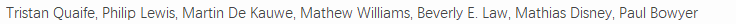``````a <- RSEDA\$Author
a
a <- unlist(strsplit(a, split = ","))
a``````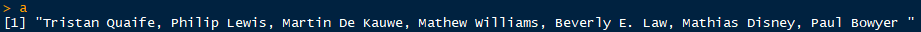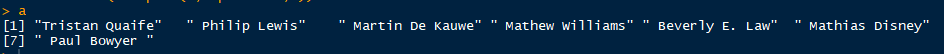``````authorlist <- list()
for (i in 1:nrow(RSEDA)) {
authors <- RSEDA\$Author[i]
authors <- unlist(strsplit(authors, split = ","))
authorlist[[i]] <- authors
}
authorlist``````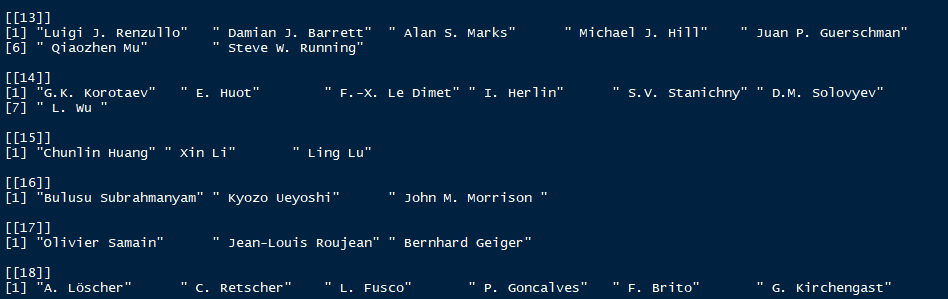``````library(stringr)
b <- " John M. Morrison "
str_trim(b, "both")``````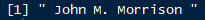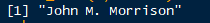``````editorlist <- list()
editorlist <- authorlist
for (i in 1:nrow(RSEDA)) {
luse <- length(authorlist[[i]])
for (m in 1:luse) {
deauthor <- authorlist[[i]][m]
deauthor <- str_trim(deauthor, 'both')
editorlist[[i]][m] <- ifelse(deauthor%in%Editor\$EditorBoard, 1, 0)
}
}``````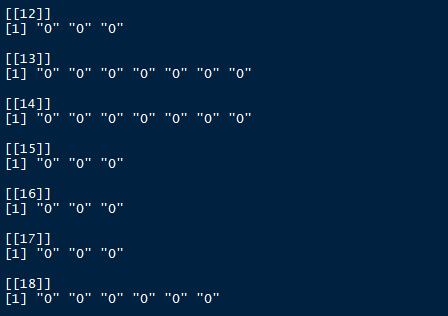``````RSEDA\$editor <- 1
for (i in 1:nrow(RSEDA)) {
RSEDA\$editor[i] <- ifelse("1"%in%editorlist[[i]], 1, 0)
}``````

+ 关注